Processing ......FreeComputerBooks.com Links to Free Computer, Mathematics, Technical Books all over the World

Nonstandard Analysis
🌠 Top Free Programming Books - 100% Free or Open Source!
• Title: Nonstandard Analysis
• Author(s) Jaap Ponstein, Isaac Goldbring
• Publisher: University of Groningen, University of California
• Hardcover: N/A
• eBook: PDF
• Language: English
• ISBN-10: 9036716721
• ISBN-13: N/A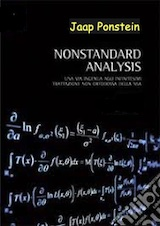Book Description

This book is concerned with an attempt to introduce the infinitesimals and the other 'nonstandard' numbers in a naive, simpleminded way. Nevertheless, the resulting theory is hoped to be mathematically sound, and to be complete within obvious limits.

• Jaap Ponstein has been a professor of Operations Research at the University of Groningen for more than 20 years. The beginning of his career in Groningen.
Reviews, Ratings, and Recommendations: Related Book Categories: Read and Download Links:Similar Books:
•Elementary Calculus: An Infinitesimal Approach (H. Jerome Keisler)

This first-year calculus book is centered around the use of infinitesimals. It contains all the ordinary calculus topics, including the basic concepts of the derivative, continuity, and the integral, plus traditional limit concepts and approximation problems.

•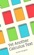Yet Another Calculus Text: A Short Introduction with Infinitesimals

An introduction to calculus based on the hyperreal number system so it uses infinitesimal and infinite numbers freely. It aims primarily at readers who already have some familiarity with calculus.

•Foundations of Infinitesimal Calculus (H. Jerome Keisler)

This monograph is a companion to the Author's textbook. It can be used as a quick introduction to the infinitesimal approach to calculus for mathematicians, as background material for instructors, or as a text for an undergraduate seminar.

•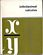Mathematical Background: Foundations of Infinitesimal

We wrote this book of foundations in part to provide a convenient reference for a student who might like to see the "theorem - proof" approach to calculus. It also shows how the pathologies arise in the traditional approach.

•Introduction to Mathematical Analysis (Beatriz Lafferriere, et al)

The goal of this book is to provide students with a strong foundation in mathematical analysis. Such a foundation is crucial for future study of deeper topics of analysis. It will be reinforced through rigorous proofs.

•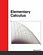Elementary Calculus (Michael Corral)

This textbook covers calculus of a single variable, is designed for students who have completed courses in high-school algebra, geometry, and trigonometry. Though designed for college students, it could also be used in high schools.

•Calculus (Gilbert Strang)

This highly regarded calculus textbook is ideal as for self-study. Examples of apps of calculus to physics, engineering and economics are included, as well as many practice questions and illustrative diagrams to assist the reader's grasp of the material.

•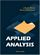Applied Analysis (John K. Hunter, et al)

This book provides an introduction to those parts of analysis that are most useful in applications for graduate students. The material is selected for use in applied problems, and is presented clearly and simply but without sacrificing mathematical rigor.

•Calculus: Theory and Applications (Kenneth Kuttler)

This is a book on single variable calculus including most of the important applications of calculus. It also includes proofs of all theorems presented, either in the text itself, or in an appendix. It also contains an introduction to vectors and vector products.

•Advanced Calculus (Lynn H. Loomis, et al)

Problem solving and mathematical modeling are introduced early and reinforced throughout, providing students with a solid foundation in the principles of mathematical thinking.

•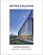Active Calculus (Matt Boelkins)

Rather than detailed explanations and worked out examples, this book uses activities intended to be done by the students in order to present the standard concepts and computational techniques of calculus.

•Calculus (WikiBooks)

This wikibook aims to be a high quality calculus textbook through which users can master the discipline. Standard topics such as limits, differentiation and integration are covered, as well as several others.

•Precalculus: An Investigation of Functions (David Lippman, et al)

Comprehensive and evenly paced, this book provides complete coverage of the function concept, and integrates a significant amount of graphing calculator material to help students develop insight into mathematical ideas.

Book Categories
 :All CategoriesTop Free BooksRecent BooksMiscellaneous BooksComputer EngineeringComputer LanguagesComputer ScienceData Science/DatabasesJava and Java EE (J2EE)Linux and UnixMathematicsMicrosoft and .NETMobile ComputingNetworking and CommunicationsSoftware EngineeringSpecial TopicsWeb Programming
Other Categories